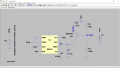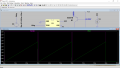# Problem with Ramp signal Generator using IC 555

#### Pr1y4n5hu

Joined Apr 21, 2022
4
According to theory the time period for ramp signal generated using the following circuit should be:
$\frac{2}{3} \frac{V_{CC}.C_{1}.R_{1}.(R_{1}+R_{2})}{V_{CC}.R_{2}-V_{BE}.(R_{1}+R_{2})}$

Now... putting the value of Vcc, R1, R2, VBE, RE... the time period comes out to be around 0.9ms.
But from the simulation it appears to be around 3ms.#### Pr1y4n5hu

Joined Apr 21, 2022
4
Please ignore the values on the hand-drawn circuit attachment. The value i calculated by formula was by taking R1,R2,Vcc,VBE, C1 same as in the spice simulation.

#### Ian0

Joined Aug 7, 2020
7,509
Does the voltage at the junction of R1 and R2 look wrong to you?
Plot it and see what it does.
Where is Re in your equation?

#### Pr1y4n5hu

Joined Apr 21, 2022
4
Does the voltage at the junction of R1 and R2 look wrong to you?
Plot it and see what it does.
Where is Re in your equation?
According to theory the time period for ramp signal generated using the following circuit should be:
$\frac{2}{3} \frac{V_{CC}.C_{1}.R_{1}.(R_{1}+R_{2})}{V_{CC}.R_{2}-V_{BE}.(R_{1}+R_{2})}$

Now... putting the value of Vcc, R1, R2, VBE, RE... the time period comes out to be around 0.9ms.
But from the simulation it appears to be around 3ms.
View attachment 266763View attachment 266764
im sorry its ...
$\frac{2}{3} \frac{V_{CC}.C_{1}.R_{E}.(R_{1}+R_{2})}{V_{CC}.R_{2}-V_{BE}.(R_{1}+R_{2})}$

#### Pr1y4n5hu

Joined Apr 21, 2022
4
Does the voltage at the junction of R1 and R2 look wrong to you?
Plot it and see what it does.
Where is Re in your equation?
im sorry its ...
$\frac{2}{3} \frac{V_{CC}.C_{1}.R_{E}.(R_{1}+R_{2})}{V_{CC}.R_{2}-V_{BE}.(R_{1}+R_{2})}$
yea it should be ~3.4V its showing 4.3V
but when i plotted it its showing a 3.4V signal

#### crutschow

Joined Mar 14, 2008
32,085
Last edited: